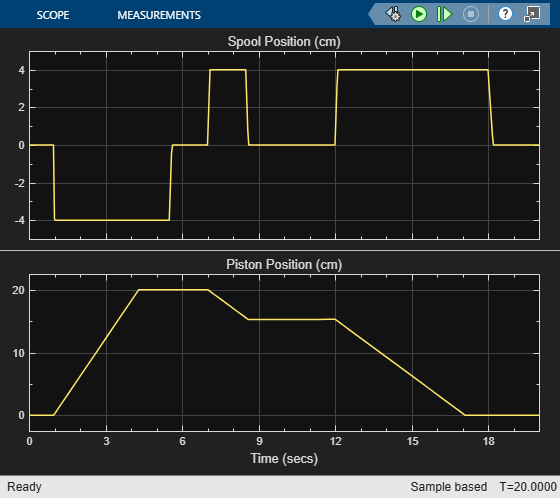# Hydraulic Actuator with Counterbalance Valve

The model consists of a double-acting hydraulic cylinder controlled by an open-center 4-way directional valve and a power unit built of a flow rate source and a pressure-relief valve. The cylinder is loaded with an active force in 10000 N trying to extend the rod. In other words, the cylinder experiences an overriding load while extending. To hold the rod in place while the directional valve is in the neutral position and prevent losing control of the cylinder extension, the counterbalance valve is used. The valve controls the return flow from port A. The valve pilot port P is connected to cylinder port B, thus making it impossible for the cylinder to move until pressure at port B builds up to certain level.

To hold the load, the counterbalance valve must be set to 1.3 * 10000/ 5e-3 = 26e5 Pa, where 1.3 is the safety coefficient, 10000 N is the load, 5e-5 m^2 is the effective piston area in the rod chamber. The cylinder starts moving as the pilot pressure builds up to approximately p = (p_s - p_L) / (k_p + k_c), where p_s is the valve setting pressure, p_L is the load pressure (p_L = L/A_r), L is the load, A_r is the rod chamber effective area (piston side B), k_p is the pilot ratio (k_p is set to 3 in the model), and k_c is the ratio between the cylinder blank chamber area to the rod chamber area ((piston area side B/piston area side A) is set to 2 in the model). As a result, the load starts moving at p = (26e5 - 100000/5e-3) / (3 + 2) = 1.2e5 Pa.

The system is simulated during a 10 second cycle, within which the rod extends by about 0.06 m and then returns to initial position. The counterbalance valve maintains enough pressure in the cylinder chamber to balance the load while the directional valve is in the neutral position.

### Model### Simulation Results from Simscape Logging

The plots below show the pressures in the cylinder and the position of the piston.The plots below show the port pressures and flow rates for the counterbalance valve. During the first part of the cycle, flow rate through the variable orifice is observed, indicating that the pressures at ports P and L create enough force to overcome the spring force and open the valve. In the second part of the cycle, flow is observed through the check valve in the counterbalance valve.